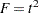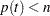PROC TRANSREG Statement
PROC TRANSREG <DATA=SAS-data-set>
<PLOTS=(plot-requests)>
<OUTTEST=SAS-data-set> <a-options> <o-options>
;

The PROC TRANSREG statement invokes the TRANSREG procedure. Optionally, this statement identifies an input and an OUTTEST= data set, specifies the algorithm and other computational details, requests displayed output, and controls the contents of the OUT= data set (which is created with the OUTPUT statement). The DATA=, OUTTEST=, and PLOTS= options can appear only in the PROC TRANSREG statement. The options listed in Table 93.1 are available in the PROC TRANSREG statement. The a-options are also available in the MODEL statement, and the o-options are also available in the OUTPUT statement.

Table 93.1 Options Available in the PROC TRANSREG Statement

Option

Description

Data Set Options (PROC Statement)

Specifies input SAS data set

Specifies output test statistics data set

ODS Graphics (PROC Statement)

Specifies ODS Graphics selection

Input Control (PROC or MODEL)

Restarts the iterations

Specifies input observation type

Method and Iterations (PROC or MODEL)

Specifies minimum criterion change

Specifies minimum data change

Specifies maximum number of iterations

Specifies iterative algorithm

Specifies number of canonical variables

Specifies no restrictions on smoothing models

Specifies singularity criterion

Attempts direct solution instead of iteration

Missing Data Handling (PROC or MODEL)

Fits each model individually (METHOD=MORALS)

Includes monotone special missing values

Excludes observations with missing values

Unties special missing values

Intercept and CLASS Variables (PROC or MODEL)

Specifies CLASS coded variable name prefix

Specifies CLASS coded variable label prefix

Specifies no intercept or centering

Specifies order of CLASS variable levels

Controls output of reference levels

Controls CLASS coded variable label separators

Control Displayed Output (PROC or MODEL)

Specifies confidence limits alpha

Displays parameter estimate confidence limits

Displays model specification details

Displays iteration histories

Suppresses displayed output

Prints the Box-Cox log likelihood table

Displays the R square

Suppresses the iteration histories

Displays regression results

Displays ANOVA table

Shortens transformed variable labels

Displays conjoint part-worth utilities

Standardization (PROC or MODEL)

Does not zero constant variables

Specifies transformation standardization

Predicted Values, Residuals, Scores (PROC or OUTPUT)

Outputs canonical scores

Outputs individual confidence limits

Outputs mean confidence limits

Specifies design matrix coding

Replaces dependent variables

Replaces independent variables

Outputs leverage

NORESTOREMISSING

Does not restore missing values

Suppresses output of scores

Outputs predicted values

Outputs redundancy variables

Replaces all variables

Outputs residuals

Output Data Set Coefficients (PROC or OUTPUT)

Outputs coefficients

Outputs ideal point coordinates

Outputs marginal means

Outputs redundancy analysis coefficients

Output Data Set Variable Name Prefixes (PROC or OUTPUT)

Specifies dependent variable approximations

Specifies independent variable approximations

Specifies canonical dependent variables

Specifies conservative individual lower CL

Specifies canonical independent variables

Specifies conservative-individual-upper CL

Specifies conservative-mean-lower CL

Specifies conservative-mean-upper CL

Specifies METHOD=MORALS untransformed dependent

Specifies liberal-individual-lower CL

Specifies liberal-individual-upper CL

Specifies liberal-mean-lower CL

Specifies liberal-mean-upper CL

Specifies predicted values

Specifies residuals

Specifies redundancy variables

Specifies transformed dependents

Specifies transformed independents

Macros Variables (PROC or OUTPUT)

Creates macro variables

Other Options (PROC or OUTPUT)

Outputs dependent and independent approximations

Outputs canonical correlation coefficients

Outputs canonical elliptical point coordinates

Outputs canonical point coordinates

Outputs approximations to transformed dependents

Outputs approximations to transformed independents

Outputs elliptical point coordinates

Outputs point coordinates

Outputs multiple regression coefficients

DATA=SAS-data-set

specifies the SAS data set to be analyzed. If you do not specify the DATA= option, PROC TRANSREG uses the most recently created SAS data set. The data set must be an ordinary SAS data set; it cannot be a special TYPE= data set.

OUTTEST=SAS-data-set

specifies an output data set to contain hypothesis tests results. When you specify the OUTTEST= option, the data set contains ANOVA results. When you specify the SS2 a-option, regression tables are also output. When you specify the UTILITIES o-option, conjoint analysis part-worth utilities are also output. For more information about the OUTTEST= data set, see the section OUTTEST= Output Data Set.

PLOTS <(global-plot-options)> <= plot-request <(options)>>
PLOTS <(global-plot-options)> <= (plot-request <(options)> <... plot-request <(options)>>)>

controls the plots produced through ODS Graphics. When you specify only one plot request, you can omit the parentheses around the plot request. Here are some examples:

```plots=none
plots=(residuals transformation)
plots(unpack)=boxcox
plots(unpack)=(transformation boxcox(p=0))
plots=(residuals(unpack) transformation(dep unp) boxcox(t rmse))
```

ODS Graphics must be enabled before requesting plots. For example:

```ods graphics on;

proc transreg plots=all;
model identity(y) = pbspline(x);
run;

ods graphics off;
```

For more information about enabling and disabling ODS Graphics, see the section Enabling and Disabling ODS Graphics in Chapter 21, Statistical Graphics Using ODS.

If ODS Graphics is enabled, but you do not specify the PLOTS= option, then PROC TRANSREG produces a default set of plots. The fit, scatter, residual, and observed-by-predicted plots are available with METHOD=MORALS and also with METHOD=UNIVARIATE when there is only one dependent variable. When no method is specified and there is more than one dependent variable, and when regression plots are requested, the default method is set to METHOD=MORALS. When there is more than one dependent variable, when METHOD= is not specified, or when METHOD=MORALS is specified and PLOTS=ALL is specified, the plots that are produced might be different from those you would see with METHOD=UNIVARIATE and PLOTS=ALL. Certain plots appear by default when ODS Graphics is enabled and certain combinations of options are specified. The Box-Coxand log-likelihood plots appear when a BOXCOX dependent variable transformation is specified. The regression fit plot appears for models with a single dependent variable that is not transformed (for example, IDENTITY(y)), a single quantitative independent variable that might or might not be transformed, and at most one CLASS independent variable. Preference mapping plots appear when the COORDINATES o-option is used.

The global plot options include the following:

INTERPOLATE
INT

uses observations that are excluded from the analysis for interpolation in the fit and transformation plots. By default, observations with zero weight are excluded from all plots. These include observations with a zero, negative, or missing weight or frequency and observations excluded due to missing and invalid values. You can specify PLOTS(INTERPOLATE)=(plot-requests) to include some of these observations in the plots. You might want to use this option, for example, with sparse data sets to show smoother functions over the range of the data (see the section The PLOTS(INTERPOLATE) Option). Observations with missing values in CLASS variables are excluded from the plots even when PLOTS(INTERPOLATE) is specified.

ONLY
ONL

suppresses the default plots. Only plots specifically requested are displayed.

UNPACKPANEL
UNPACK
UNP

suppresses paneling. By default, multiple plots can appear in some output panels. Specify UNPACKPANEL to get each plot in a separate panel. You can specify PLOTS(UNPACKPANEL) to unpack the default plots. You can also specify UNPACKPANEL as a suboption with TRANSFORMATION, RESIDUALS, PBSPLINE, and BOXCOX.

The plot requests include the following:

ALL

produces all appropriate plots. You can specify other options with ALL; for example, to request all plots and unpack only the residuals, specify PLOTS=(ALL RES(UNP)).

BOXCOX <( options )>
BOX <( options )>

requests a display of the results of the Box-Cox transformation. These results are displayed by default when there is a Box-Cox transformation. The BOXCOX plot request has the following options:

P=n

adds t orcurves to the legend for the functions where, where t is the t statistic corresponding to the optimal lambda. You can specify P=0 to suppress the legend and P=1 to see all curves in the legend. The default value comes from the BOXCOX(variable / ALPHA=p) specification, which by default is 0.05.

RMSE
RMS

plots the root mean square error as a function of lambda.

T

plots t statistics rather thanstatistics.

UNPACKPANEL
UNPACK
UNP

plots the t orand log-likelihood plots in separate panels.

FIT <( options )>

requests a regression fit plot. This plot is produced by default whenever it is appropriate. It is produced when the dependent variable is specified with the IDENTITY transform, and when there is one quantitative independent variable (for example, IDENTITY for linear fit or SPLINE or one of the other transformations for a nonlinear fit) and at most one CLASS variable. When there is a CLASS variable, separate fits are produced within levels based on your model. You would specify the FIT plot request only to specify a FIT option or with the ONLY global plot option. The FIT plot request has the following options:

NOCLM

suppresses the confidence limits in regression fit plots.

NOCLI

suppresses the individual prediction limits in regression fit plots.

NOOBS

suppresses the observations showing only the fit function and optionally the confidence and prediction limits.

NONE

suppresses all plots.

OBSERVEDBYPREDICTED
OBP
OBS

plots the transformed dependent variable as a function of the regression predicted values.

PBSPLINE <( UNPACKPANEL )>
PBS <( UNPACK )>

requests the penalized B-spline criterion plots. You would specify the PBSPLINE plot request only to specify a PBSPLINE option or with the ONLY global plot option. The PBSPLINE plot request has the following option:

UNPACKPANEL
UNPACK
UNP

plots each criterion plot in a separate panel.

PREFMAP
PRE

plots ideal point or vector preference mapping results when either two IDENTITY or two POINT independent variables are specified along with the COORDINATES option.

RESIDUALS <( options )>
RES <( options )>

plots the residuals as a function of each of the transformed independent variables, except coded CLASS variables. The RESIDUALS plot request has the following options:

CLASS
CLA

plots the residuals as a function of each of the transformed independent variables, including coded CLASS variables. Note that the ALL plot request, which you use to request all plots, specifies the RESIDUALS plot request without the CLASS option.

UNPACKPANEL
UNPACK
UNP

plots the residuals in separate plots, not several per panel.

SMOOTH
SMO

adds a LOESS smooth function to the residuals plots.

SCATTER
SCA

plots the scatter plot of observed data, before the transformations, for models with a single quantitative dependent variable, a single quantitative independent variable, and at most one CLASS independent variable.

TRANSFORMATION <( options )>
TRA <( options )>

plots the variable transformations. The TRANSFORMATION plot request has the following options:

DEPENDENTS
DEP

plots only the dependent variable transformations.

INDEPENDENTS
IND

plots only the independent variable transformations.

UNPACKPANEL
UNPACK
UNP

plots the transformations in separate plots, not several per panel.